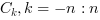Scilab Home page | Wiki | Bug tracker | Forge | Mailing list archives | ATOMS | File exchange
Change language to: English - Français - 日本語 - Русский

Please note that the recommended version of Scilab is 6.0.2. This page might be outdated.
See the recommended documentation of this function

xcorr

Computes discrete auto or cross correlation

Calling Sequence

[c [,lagindex]] = xcorr(x [,maxlags [,scaling]])
[c [,lagindex]] = xcorr(x,y [,maxlags [,scaling]])

Parameters

x

a vector of real or complex floating point numbers.

y

a vector of real or complex floating point numbers. The default value is x.

maxlags

a scalar with integer value greater than 1. The default value is n. Where n is the maximum of the x and y vector length.

scaling

a character string with possible value: "biased", "unbiased", "coeff", "none". The default value is "none".

c

a vector of real or complex floating point numbers with same orientation as x.

lagindex

a row vector, containing the lags index corresponding to the c values.

Description

• c=xcorr(x) computes the un-normalized discrete auto correlation:and return in c the sequence of auto correlation lagswith n is the length of x
• xcorr(x,y) computes the un-normalized discrete cross correlation:and return in c the sequence of auto correlation lagswith n is the maximum of x and y length's.

If the maxlags argument is given xcorr returns in c the sequence of auto correlation lags. If maxlags is greater than length(x), the first and last values of c are zero.

The scaling argument decribes howis normalized before being returned in c:

• "biased":c=/n.
• "unbiased":c=./(n-(-maxlags:maxlags)).
• "coeff":c=/(norm(x)*norm(y)).

Remark

The corr function computes the "biased" covariance of x and y and only return in c the sequence of auto correlation lags.

Method

This function computesusing ifft(fft(x).*conj(fft(y))).

Examples

t = linspace(0, 100, 2000);
y = 0.8 * sin(t) + 0.8 * sin(2 * t);
[c, ind] = xcorr(y, "biased");
plot(ind, c)Authors

• Serge Steer, INRIA

fft

History

 Versão Descrição 5.4.0 xcorr added.